JEE  >  Irodov Solutions: Transport Phenomena- 2

# Irodov Solutions: Transport Phenomena- 2 | I. E. Irodov Solutions for Physics Class 11 & Class 12 - JEE

 1 Crore+ students have signed up on EduRev. Have you?

Q. 239. An ideal gas goes through a polytropic process. Find the polytropic exponent n if in this process the coefficient
(a) of diffusion;
(b) of viscosity;
(c) of heat conductivity remains constant.

Solution. 239.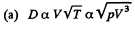Thus D remains constant in the process pV3 = constant

So polytropic index n = 3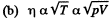So η remains constant in the isothermal process

pV = constant, n = 1, here

(c) Heat conductivity k = ηCv and Cv is a constant for the ideal gas

Thus n = 1 here also,

Q. 240. Knowing the viscosity coefficient of helium under standard conditions, calculate the effective diameter of the helium atom.

Solution. 240.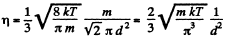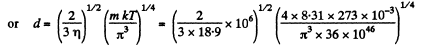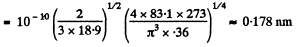Q. 241. The heat conductivity of helium is 8.7 times that of argon (under standard conditions). Find the ratio of effective diameters of argon and helium atoms.

Solution. 241.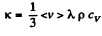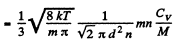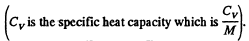Now Cv is the same for all monoatomic  gases such as He and A. Thus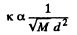or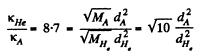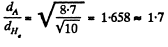Q. 242. Under standard conditions helium fills up the space between two long coaxial cylinders. The mean radius of the cylinders is equal to R, the gap between them is equal to ΔR, with AR ≪ R. The outer cylinder rotates with a fairly low angular velocity o about the stationary inner cylinder. Find the moment of friction forces acting on a unit length of the inner cylinder. Down to what magnitude should the helium pressure be lowered (keeping the temperature constant) to decrease the sought moment of friction forces n = 10 times if ΔR = 6 mm?

Solution. 242. In this case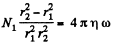or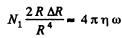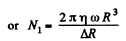To decrease N1,n times η must be decreased n times. Now η does not depend on pressure until the pressure is so low that the mean free path equals,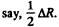Then the mean free path is fixed and η  decreases with pressure. The mean free path equals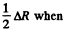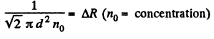Corresponding pressure is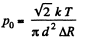The sought pressure is n times less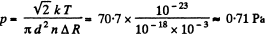The answer is qualitative and depends on die choice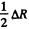for the mean free path.

Q. 243. A gas fills up the space between two long coaxial cylinders of radii R1 and R2, with R1 < R2. The outer cylinder rotates with a fairly low angular velocity ω about the stationary inner cylinder. The moment of friction forces acting on a unit length of the inner cylinder is equal to N1. Find the viscosity coefficient η of the gas taking into account that the friction force acting on a unit area of the cylindrical surface of radius r is determined by the formula σ =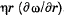Solution. 243. We neglect the moment of inertia of the gas in a shell. Then the moment of friction forces on a unit length of the cylinder must be a constant as a function of r.

So,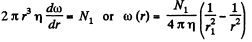and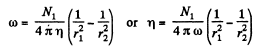Q. 244. Two identical parallel discs have a common axis and are located at a distance h from each other. The radius of each disc is equal to a, with a ≫ h. One disc is rotated with a low angular velocity ω relative to the other, stationary, disc. Find the moment of friction forces acting on the stationary disc if the viscosity coefficient of the gas between the discs is equal to η

Solution. 244. We consider two adjoining layers. The angular velocity gradient is ω/h. So the moment of the frictional force is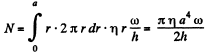Q. 245.  Solve the foregoing problem, assuming that the discs are located in an ultra-rarefied gas of molar mass M, at temperature T and under pressure p.

Solution. 245. In the ultrararefled gas we must determine η by taking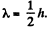Then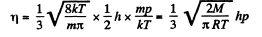so,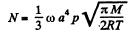Q. 246. Making use of Poiseuille's equation (1.7d), find the mass μ of gas flowing per unit time through the pipe of length l and radius a if constant pressures p1  and p2 are maintained at its ends.

Solution. 246. Take an infinitesimal section of length dx and apply Poiseuilles equation to this. Then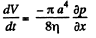From the formula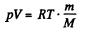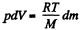or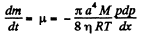This equation implies that if the flow is isothermal then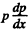must be a constant and so equals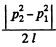in magnitude.

Thus,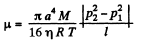Q. 247. One end of a rod, enclosed in a thermally insulating sheath, is kept at a temperature T1 while the other, at T2. The rod is composed of two sections whose lengths are l1 and l2 and heat conductivity coefficients x1 and x2. Find the temperature of the interface.

Solution. 247. Let T = temperature of the interface.

Then heat flowing from left = heat flowing into right in equilibrium.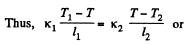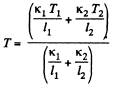Q. 248. Two rods whose lengths are l1 and 12 and heat conductivity coefficients x1 and x2  are placed end to end. Find the heat conductivity coefficient of a uniform rod of length l1 + l2 whose conductivity is the same as that of the system of these two rods. The lateral surfaces of the rods are assumed to be thermally insulated.

Solution. 248. We have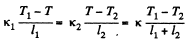or using the previous result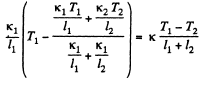or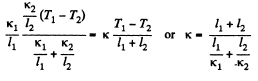Q. 249. A rod of length l with thermally insulated lateral surface consists of material whose heat conductivity coefficient varies with temperature as x = α/T, where α is a constant. The ends of the rod are kept at temperatures T1 and T2. Find the function T (x), where x is the distance from the end whose temperature is T1, and the heat flow density

Solution. 249. By definition the heat flux (per unit area) is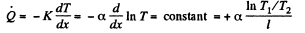Integrating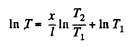where T1 =  temperature at the end x = 0

So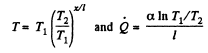Q. 250. Two chunks of metal with heat capacities C1 and C2 are interconnected by a rod of length l and cross-sectional area S and fairly low heat conductivity x. The whole system is thermally insulated from the environment. At a moment t = 0 the temperature difference between the two chunks of metal equals (ΔT)0. Assuming the heat capacity of the rod to be negligible, find the temperature difference between the chunks as a function of time.

Solution. 250. Suppose the chunks have temperatures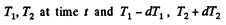at time dt + t.

Then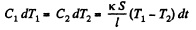Thus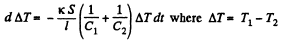Hence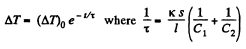Q. 251. Find the temperature distribution in a substance placed between two parallel plates kept at temperatures T1 and T2. The plate separation is equal to 1, the heat conductivity coefficient of the substance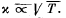Solution. 251.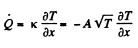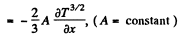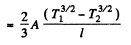Thus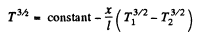or using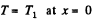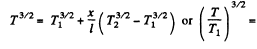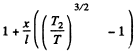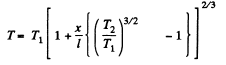Q. 252. The space between two large horizontal plates is filled with helium. The plate separation equals l = 50 mm. The lower plate is kept at a temperature T1 = 290 K, the upper, at T2 = 330 K. Find the heat flow density if the gas pressure is close to standard.

Solution. 252.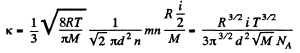Then from the previous problem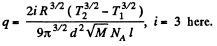Q. 253. The space between two large parallel plates separated by a distance l = 5.0 mm is filled with helium under a pressure p = 1.0 Pa. One plate is kept at a temperature t1 = 17°C and the other, at a temperature t2 = 37°C. Find the mean free path of helium atoms and the heat flow density.

Solution. 253. At this pressure and average temparature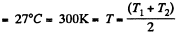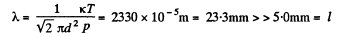The gas is ultrathin and we write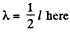Then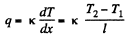where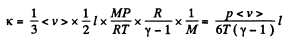and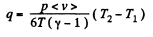where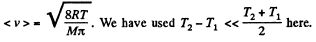Q. 254. Find the temperature distribution in the space between two coaxial cylinders of radii R1 and R2  filled with a uniform heat conducting substance if the temperatures of the cylinders are constant and are equal to T1  and T2 respectively.

Solution. 254. In equilibrium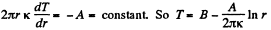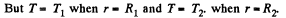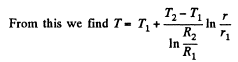Q. 255. Solve the foregoing problem for the case of two concentric spheres of radii. R1 and R2 and temperatures T1 and T2

Solution. 255. In equilibrium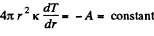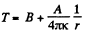Using T - T1 when r = R1 and T = T2 when r = R2,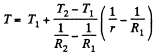Q. 256. A constant electric current flows along a uniform wire with cross-sectional radius R and heat conductivity coefficient x. A unit volume of the wire generates a thermal power w. Find the temperature distribution across the wire provided the steady-state temperature at the wire surface is equal to T0.

Solution. 256.  The heat flux vector is - k grad T and its divergence equals w. Thus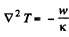or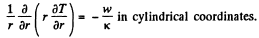or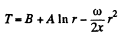Since T is finite at r = 0, A = 0. Also T = T0 at r = R

so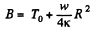Thus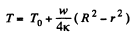r here is the distance from the axis of wire (axial radius).

Q. 257. The thermal power of density w is generated uniformly inside a uniform sphere of radius R and heat conductivity coefficient x. Find the temperature distribution in the sphere provided the steady-state temperature at its surface is equal to T0

Solution. 257.  Here again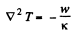So in spherical polar coordinates,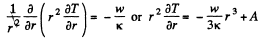or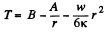Again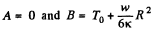so finally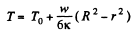The document Irodov Solutions: Transport Phenomena- 2 | I. E. Irodov Solutions for Physics Class 11 & Class 12 - JEE is a part of the JEE Course I. E. Irodov Solutions for Physics Class 11 & Class 12.
All you need of JEE at this link: JEE

111 docs

## I. E. Irodov Solutions for Physics Class 11 & Class 12

111 docs

Track your progress, build streaks, highlight & save important lessons and more!(Scan QR code)

,

,

,

,

,

,

,

,

,

,

,

,

,

,

,

,

,

,

,

,

,

;